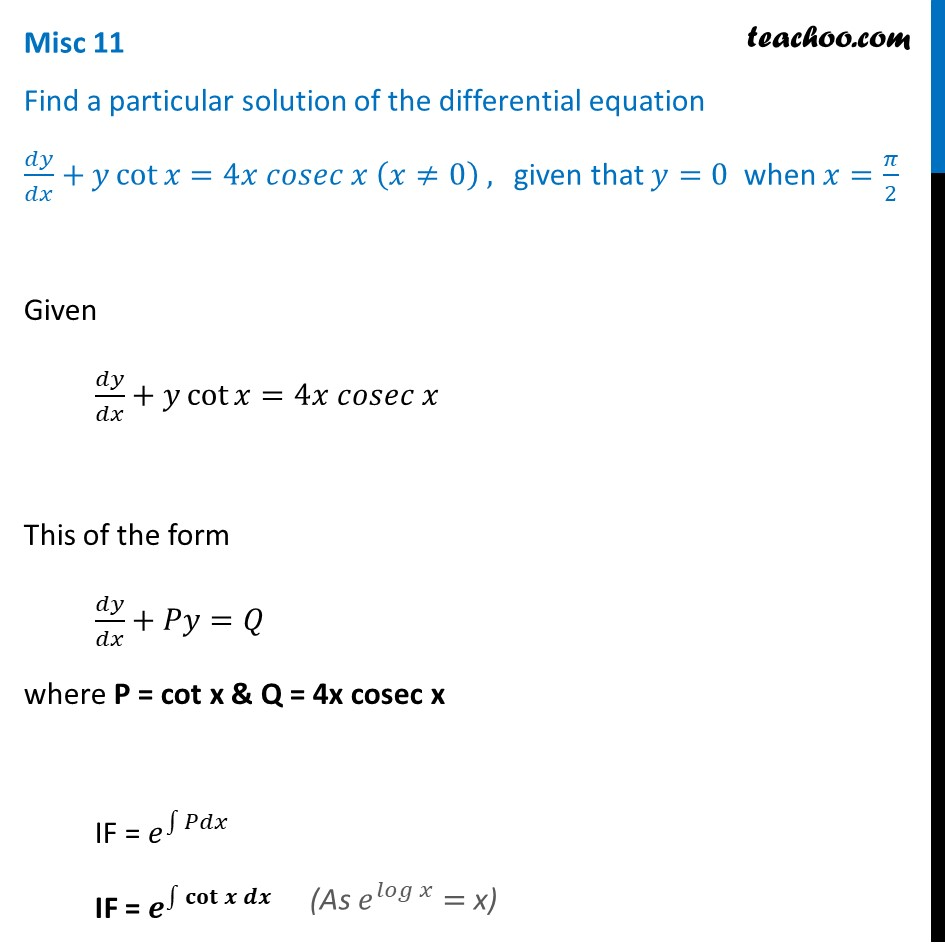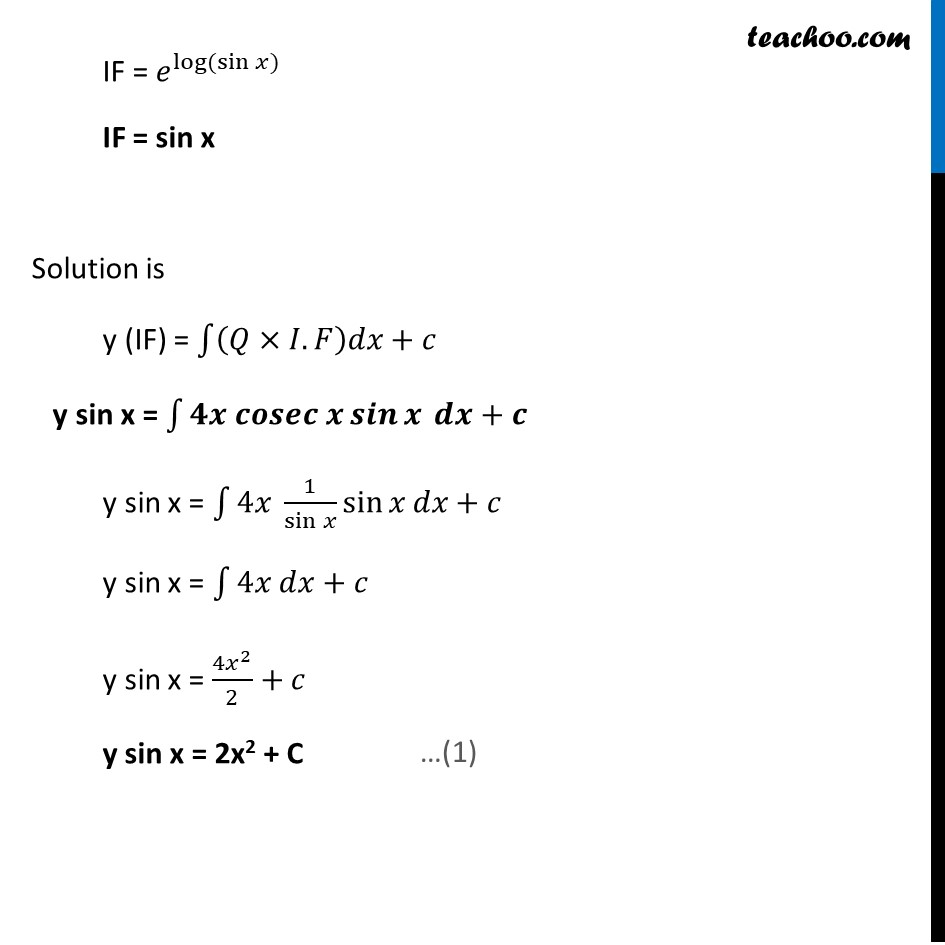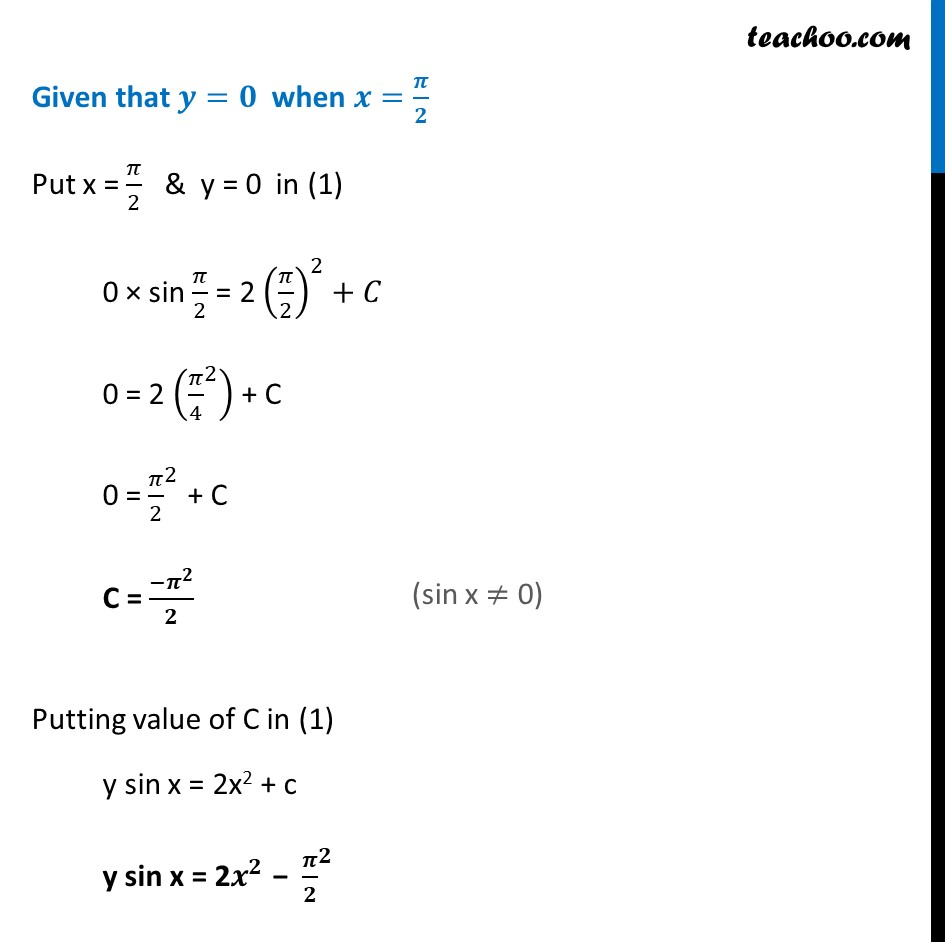Miscellaneous

Chapter 9 Class 12 Differential Equations
Serial order wiseLearn in your speed, with individual attention - Teachoo Maths 1-on-1 Class

### Transcript

Misc 11 Find a particular solution of the differential equation 𝑑𝑦/𝑑𝑥+𝑦 cot⁡〖𝑥=4𝑥 𝑐𝑜𝑠𝑒𝑐 𝑥 (𝑥≠0) ,〗 given that 𝑦=0 when 𝑥=𝜋/2Given 𝑑𝑦/𝑑𝑥+𝑦 cot⁡〖𝑥=4𝑥 𝑐𝑜𝑠𝑒𝑐 𝑥 〗 This of the form 𝑑𝑦/𝑑𝑥+𝑃𝑦=𝑄 where P = cot x & Q = 4x cosec x IF = 𝑒^∫1▒𝑃𝑑𝑥 IF = 𝒆^∫1▒𝐜𝐨𝐭⁡〖𝒙 𝒅𝒙〗 IF = 𝑒^(log⁡(sin⁡〖𝑥)〗 ) IF = sin x Solution is y (IF) = ∫1▒〖(𝑄×𝐼.𝐹)𝑑𝑥+𝑐 〗 y sin x = ∫1▒〖𝟒𝒙 𝒄𝒐𝒔𝒆𝒄 𝒙 𝒔𝒊𝒏⁡𝒙 𝒅𝒙+𝒄 〗 y sin x = ∫1▒〖4𝑥 1/sin⁡𝑥 sin⁡𝑥 𝑑𝑥+𝑐 〗 y sin x = ∫1▒〖4𝑥 𝑑𝑥+𝑐 〗 y sin x = (4𝑥^2)/2+𝑐 y sin x = 2x2 + C Given that 𝒚=𝟎 when 𝒙=𝝅/𝟐 Put x = 𝜋/2 & y = 0 in (1) 0 × sin 𝜋/2 = 2 (𝜋/2)^2+𝐶 0 = 2 (〖𝜋/4〗^2 ) + C 0 = 〖𝜋/2〗^2 + C C = 〖−𝝅〗^𝟐/𝟐 Putting value of C in (1) y sin x = 2x2 + c y sin x = 2𝒙^𝟐 − 〖𝝅/𝟐〗^𝟐